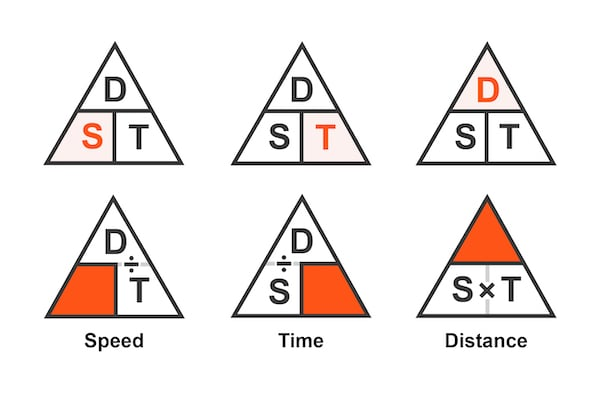# Speed Distance Time Calculator

## This calculator will solve for either distance, time, or speed given two of the three parameters.

### Calculate Time from Distance and Speed

The relationship between an object's speed of travel, distance traveled, and time traveled is given by the following equation:

$$s = \frac{d}{t}$$

Given two of the three parameters, the calculator will determine the third value using the relationships shown in Figure 1. This can be useful when modeling physical systems such as a moving vehicle, an autonomous mobile robot (AMR), or even electron propagation through a wire.### Speed Distance Time Equation:

As noted above, to convert between values of time, speed, and distance, we use the following standard equation:

$$s = \frac{d}{t}$$

Where:

• s = The speed of the object expressed in units of distance over time
• d = The distance an object has traveled in a given period of time
• t = The time during which the object traveled a given distance

From here, we can rearrange the equation to solve for time:

$$t = \frac{d}{s}$$

Or we can solve for distance using the following equation:

$$d = s \cdot t$$